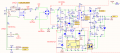# calculate flyback converter transformer current help

#### Benengineer

Joined Feb 6, 2016
133
Hi Guys,
I have questions how to figure out flyback transformer current. Please see the circuit.we know flyback converter output voltage is 24V and load current 150mA. I try to find the primary and the other two secondaries output. The transformer data sheet is in an attachments. There are three windings and one primary wind.
View attachment 105823

The winding 1 and winding 4 ration is 118/30, and winding 1 and winding 3 ration is 118/14, so does w1 and w5 ratio. The below is my calculation process.
Based on the flyback transformer circuit, we know that the current limit is I₄ = 0.7V/R63 (1.5Ω) = 0.467A

I₁ = (N₄/N₁)* I₄ = (30/118)*0.467A = 0.119A

I₃ = (N₁/N₃)* I₁ = (118/14)*0.119 = 1.003A

I₅ = I₃

Magnetizing current in max: Im = I₄ + (N₃/ N₄)* I₃ + (N₅/ N₄)* I₅ = 2.125A.

D is duty cycle, Ts is switch period. D = 0.15, Ts = 1/100K (those data were measured by me)

Δi = VᵢDTs/2*Lm = 370*2*0.15/2* (1.25x10^-3)* 100000 = 0.314A

The peak current is a little high, isn’t it?
Im. Max = Im + Δi = 2.125A + 0.314 A = 2.439A This is magnetizing current in total.
Please let me know if these calculation is right.
Thanks

#### Benengineer

Joined Feb 6, 2016
133
Hi Guys,
I have questions how to figure out flyback transformer current. Please see the circuit.
View attachment 105833
we know flyback converter output voltage is 24V and load current 150mA. I try to find the primary and the other two secondaries output. The transformer data sheet is in an attachments. There are three windings and one primary wind.
View attachment 105823

The winding 1 and winding 4 ration is 118/30, and winding 1 and winding 3 ration is 118/14, so does w1 and w5 ratio. The below is my calculation process.
Based on the flyback transformer circuit, we know that the current limit is I₄ = 0.7V/R63 (1.5Ω) = 0.467A

I₁ = (N₄/N₁)* I₄ = (30/118)*0.467A = 0.119A

I₃ = (N₁/N₃)* I₁ = (118/14)*0.119 = 1.003A

I₅ = I₃

Magnetizing current in max: Im = I₄ + (N₃/ N₄)* I₃ + (N₅/ N₄)* I₅ = 2.125A.

D is duty cycle, Ts is switch period. D = 0.15, Ts = 1/100K (those data were measured by me)

Δi = VᵢDTs/2*Lm = 370*2*0.15/2* (1.25x10^-3)* 100000 = 0.314A

The peak current is a little high, isn’t it?
Im. Max = Im + Δi = 2.125A + 0.314 A = 2.439A This is magnetizing current in total.
Please let me know if these calculation is right.
Thanks
Sorry forget to attach transformer data sheet

#### Attachments

• 428.5 KB Views: 3